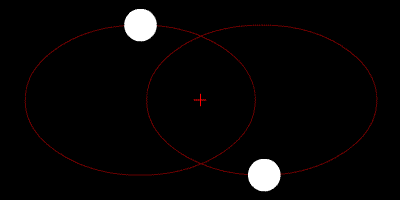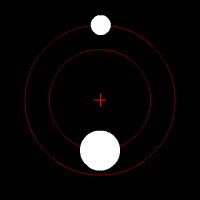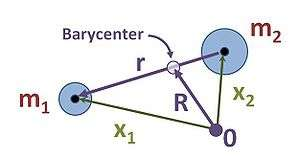# Two-body problemLeft: Two bodies with similar mass orbiting a common barycenter external to both bodies, with elliptic orbits—typical of binary stars. Right: Two bodies with a "slight" difference in mass orbiting a common barycenter. The sizes, and this type of orbit are similar to the Pluto–Charon system (in which the barycenter is external to both bodies), and to the EarthMoon system—where the barycenter is internal to the larger body.

In classical mechanics, the two-body problem is to determine the motion of two point particles that interact only with each other. Common examples include a satellite orbiting a planet, a planet orbiting a star, two stars orbiting each other (a binary star), and a classical electron orbiting an atomic nucleus (although to solve the electron/nucleus 2-body system correctly a quantum mechanical approach must be used).

The two-body problem can be re-formulated as two one-body problems, a trivial one and one that involves solving for the motion of one particle in an external potential. Since many one-body problems can be solved exactly, the corresponding two-body problem can also be solved. By contrast, the three-body problem (and, more generally, the n-body problem for n  3) cannot be solved in terms of first integrals, except in special cases.

## Reduction to two independent, one-body problemsJacobi coordinates for two-body problem; Jacobi coordinates areandwith.

Let x1 and x2 be the vector positions of the two bodies, and m1 and m2 be their masses. The goal is to determine the trajectories x1(t) and x2(t) for all times t, given the initial positions x1(t = 0) and x2(t = 0) and the initial velocities v1(t = 0) and v2(t = 0).

When applied to the two masses, Newton's second law states thatwhere F12 is the force on mass 1 due to its interactions with mass 2, and F21 is the force on mass 2 due to its interactions with mass 1. The two dots on top of the x position vectors denote their second derivative, or their acceleration vectors.

Adding and subtracting these two equations decouples them into two one-body problems, which can be solved independently. Adding equations (1) and (2) results in an equation describing the center of mass (barycenter) motion. By contrast, subtracting equation (2) from equation (1) results in an equation that describes how the vector r = x1  x2 between the masses changes with time. The solutions of these independent one-body problems can be combined to obtain the solutions for the trajectories x1(t) and x2(t).

### Center of mass motion (1st one-body problem)

Addition of the force equations (1) and (2) yieldswhere we have used Newton's third law F12 = F21 and whereis the position of the center of mass (barycenter) of the system.

The resulting equation:shows that the velocity V = dR/dt of the center of mass is constant, from which follows that the total momentum m1 v1 + m2 v2 is also constant (conservation of momentum). Hence, the position R (t) of the center of mass can be determined at all times from the initial positions and velocities.

## Two-body motion is planar

The motion of two bodies with respect to each other always lies in a plane (in the center of mass frame). Defining the linear momentum p and the angular momentum L by the equations (where μ is the reduced mass)the rate of change of the angular momentum L equals the net torque Nand using the property of the vector cross product that v × w = 0 for any vectors v and w pointing in the same direction,with F = μ d 2r / dt 2.

Introducing the assumption (true of most physical forces, as they obey Newton's strong third law of motion) that the force between two particles acts along the line between their positions, it follows that r × F =  0 and the angular momentum vector L is constant (conserved). Therefore, the displacement vector r and its velocity v are always in the plane perpendicular to the constant vector L.

## Energy of the two-body system

If the force F(r) is conservative then the system has a potential energy U(r), so the total energy can be written asIn the center of mass frame the kinetic energy is the lowest and the total energy becomesThe coordinates x1 and x2 can be expressed asand in a similar way the energy E is related to the energies E1 and E2 that separately contain the kinetic energy of each body:## Central forces

For many physical problems, the force F(r) is a central force, i.e., it is of the formwhere r = |r| and = r/r is the corresponding unit vector. We now have:where F(r) is negative in the case of an attractive force.

## Work

The total work done in a given time interval by the forces exerted by two bodies on each other is the same as the work done by one force applied to the total relative displacement.